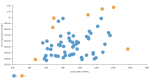# DCPY.ISOLATIONFOREST(n_estimators, max_samples, max_features, contamination, columns)

Isolation Forest is a multivariate outlier detection algorithm that isolates observations by randomly selecting a feature, and then randomly selecting a split value between the maximum and minimum values of the selected feature. It is built on an ensemble of binary (isolation) trees. Random partitioning produces noticeably shorter paths for anomalous points. When a forest of random trees collectively produce shorter path lengths for particular samples, they are highly likely be anomalous. In other words, an anomaly score can be calculated as the number of conditions required to separate a given observation.

###### Parameters
• n_estimators – Number of trees in the ensemble model, integer (default 100)

• max_samples – Number of samples to draw from the data to train each tree (default 256):

• If integer, then this number will be used to draw n samples to train each tree.
• If float, then this proportion of data will be sampled to train each tree.
• If the number is larger than the number of samples provided, all samples will be sed for all trees, so without sampling.
• max_features – Number of features to draw from data to train each tree (default 1.0):

• If integer, then this number of features will be used to train each tree.
• If float, then this proportion of features will be used to train each tree.
• contamination – Approximate proportion of outliers in the dataset, which is used as a threshold for the decision function, float (0;1) (default 0.1)

• columns – Dataset columns or custom calculations.

Example: DCPY.ISOLATIONFOREST(100, 256, 1.0, 0.1, sum([Gross Sales]), sum([No of customers])) used as a calculation for the Color field of the Scatterplot visualization.###### Input data
• Numeric variables are automatically scaled to zero mean and unit variance.

• Character variables are transformed to numeric values using one-hot encoding.

• Dates are treated as character variables, so they are also one-hot encoded.

• Size of input data is not limited, but many categories in character or date variables increase rapidly the dimensionality.

• Rows that contain missing values in any of their columns are dropped.

###### Result
• Column of values 1 corresponding to inlier, and -1 corresponding to outlier.
• Rows that were dropped from input data due to containing missing values have missing value instead of assigned inlier/outlier value.
###### Key usage points
• Like Local Outlier Factor, it performs well on multimodal data.
• Suitable for big datasets and many variables.
• Linear time complexity.
• Small memory requirements.
###### Example

For the whole list of algorithms, see Data science built-in algorithms.